# Using Mockito in Android Unit Testing as a ProMost of the classes have some dependencies and often times methods delegates some of the work to other methods in other classes, and we call these classes dependencies.

When unit testing such methods, if we only used JUnit, our tests will also depend on those methods as well. We want the unit tests to be independent of all other dependencies.

That is the reason we just Mock the dependency class and we can test the Main Class.

##### What is mockito?
Mockito is a mocking framework that tastes really good. It lets you write beautiful tests with a clean & simple API. Mockito doesn’t give you hangover because the tests are very readable and they produce clean verification errors.

Now, let us understand how to use mockito with example,

``````testImplementation 'junit:junit:4.12'
testImplementation 'org.mockito:mockito-core:2.19.0'``````

and in the java folder we will create a package called calculator having two files Operators and Calculator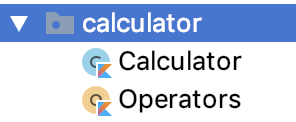Here, Operators is a object which has set of functions of calculator operations

``````object Operators {
fun add(a: Int, b: Int): Int = a + b

fun subtract(a: Int, b: Int): Int = a - b

fun multiply(a: Int, b: Int): Int = a * b

fun divide(a: Int, b: Int): Int = a / b
}``````

and Calculator is a class,

``````class Calculator(private val operators: Operators) {

fun subtractTwoNumbers(a: Int, b: Int): Int = operators.subtract(a, b)

fun multiplyTwoNumbers(a: Int, b: Int): Int = operators.multiply(a, b)

fun divideTwoNumbers(a: Int, b: Int): Int = operators.divide(a, b)
}``````

Here, Calculator takes operator object as a parameter in the primary constructor. So, we return operator function as return param for the functions in Calculator class.

#### Let's start testing the Calculator Class,

Inside the test folder, we will create a package called calculator same as we did in the java folder.

In that, we will create only one class called CalculatorTest.kt.Here, you can see we have not created OperatorsTest as we have to test only CalculatorTest here.

So, in Calulator Test,

``````@RunWith(MockitoJUnitRunner::class)
class CalculatorTest {

}``````

Here, we have annotated with MockitoJUnitRunner::class that means it provides the Runner to run the test.

Now, we will setup the calculator class

``````@RunWith(MockitoJUnitRunner::class)
class CalculatorTest {

lateinit var calculator: Calculator

@Before
fun onSetup() {
calculator = Calculator(/** opearators **/)
}
}``````

Here, in construct we need to pass the operators. So, we won't create an object of the operator as,

• We want to perform the test in isolation so that even if the Operators crashes, it should not impact the test being performed in CalculatorTest.
• We have to just have the invoke methods of the Operator class and do external communication.

Also, @Before means, that even before performing the test we need to setup the dependency.

So, here we have to mock the operator like,

``````@RunWith(MockitoJUnitRunner::class)
class CalculatorTest {

@Mock
lateinit var operators: Operators

lateinit var calculator: Calculator

@Before
fun onSetup() {
calculator = Calculator(operators)
}
}``````

In Mockito, we mock any class using @Mock annotation.

By Mocking any class, we are creating an mock object of that speicifc class. In the above code, Operators is mocked to provide dependency for Calculator class.

Now, lets perform some test now,

``````@Test
val a = 10
val b = 20

}``````

Here, to perform test we need to annotate the function with @Test.

Now, we will create two variables, a and b with values 10,20 and then we will call,

`` calculator.addTwoNumbers(a, b)``

and to verify if the correct function was called or not from the mocked class, we will just use,

`` verify(operators).add(a, b) ``

Here, verify means that you want to check if a certain method of a mock object has been called or not.

Now, to run the test we can right click on the function like,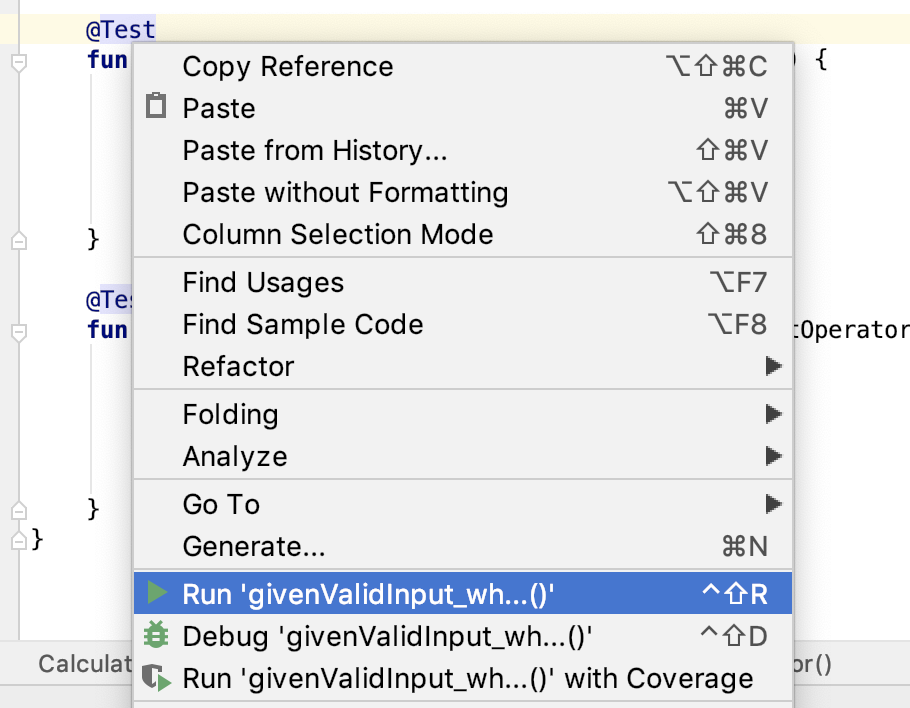and just click Run .

This will run the test and will generate the output that if the Test passed or failed. In our case, the test passed as we called the correct function.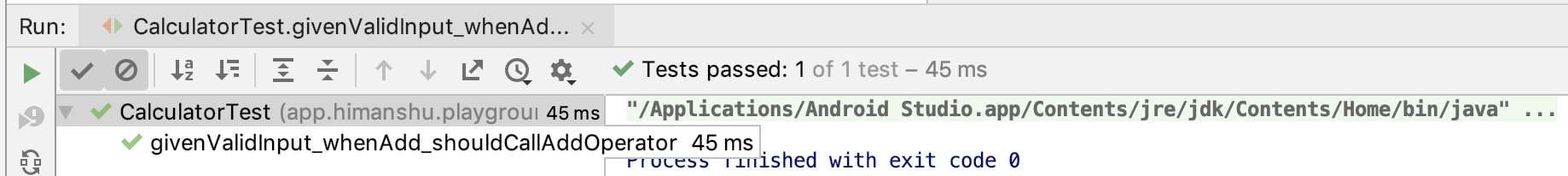Now, to fail the case, just replace

``verify(operators).subtract(a, b)``

and our test will fail as we are calling addTwoNumbers of Calculator class and then from mocked Operators we are calling subtract.

It will throw an error like below,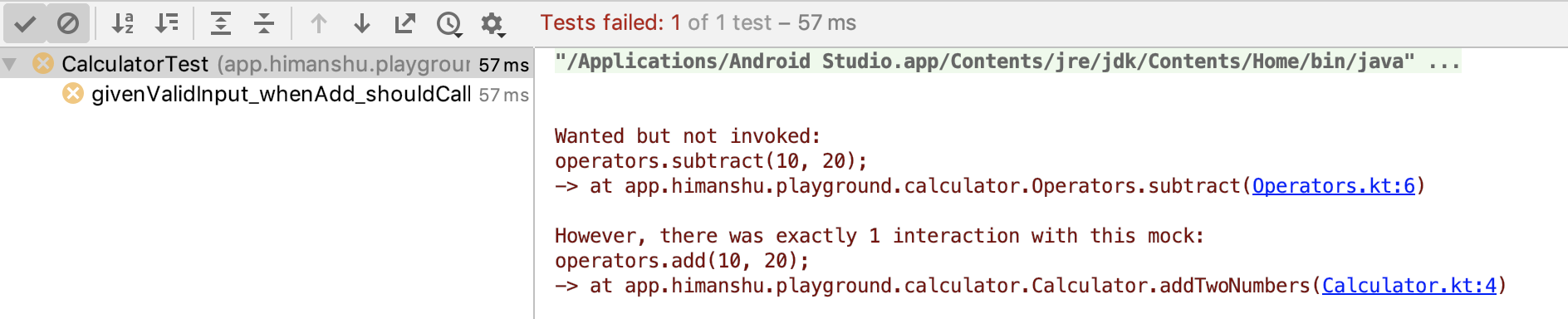This is how we can use Mockito and perform unit testing in our app.

Now, to run test on all the functions,

``````package app.himanshu.playground.calculator

import org.junit.Before
import org.junit.Test
import org.junit.runner.RunWith
import org.mockito.Mock
import org.mockito.Mockito.verify
import org.mockito.junit.MockitoJUnitRunner

@RunWith(MockitoJUnitRunner::class)
class CalculatorTest {

@Mock
lateinit var operators: Operators

lateinit var calculator: Calculator

@Before
fun onSetup() {
calculator = Calculator(operators)
}

@Test
val a = 10
val b = 20

}

@Test
fun givenValidInput_whenSubtract_shouldCallSubtractOperator() {
val a = 10
val b = 20
calculator.subtractTwoNumbers(a, b)
verify(operators).subtract(a, b)

}
@Test
fun givenValidInput_whenMultiply_shouldCallMultiplyOperator() {
val a = 10
val b = 20
calculator.multiplyTwoNumbers(a, b)
verify(operators).multiply(a, b)

}
@Test
fun givenValidInput_whenDivide_shouldCallDivideOperator() {
val a = 10
val b = 20
calculator.divideTwoNumbers(a, b)
verify(operators).divide(a, b)

}
}``````

and to run all the test we can just Right Click on CalculatorTest -> Run CalculatorTest. It will run all the test at once and genetate the following success output.,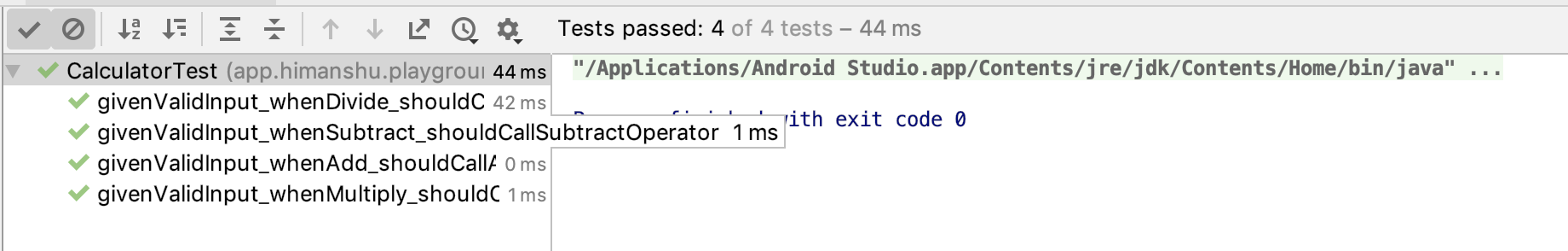Note : As all classes in Kotlin are final, Mockito can't directly test the final/static classes. To run test on final classes we need to setup the mockito-extentions. You can read more about it here.

Happy learning

Team MindOrks :)